Steam Paathshaala English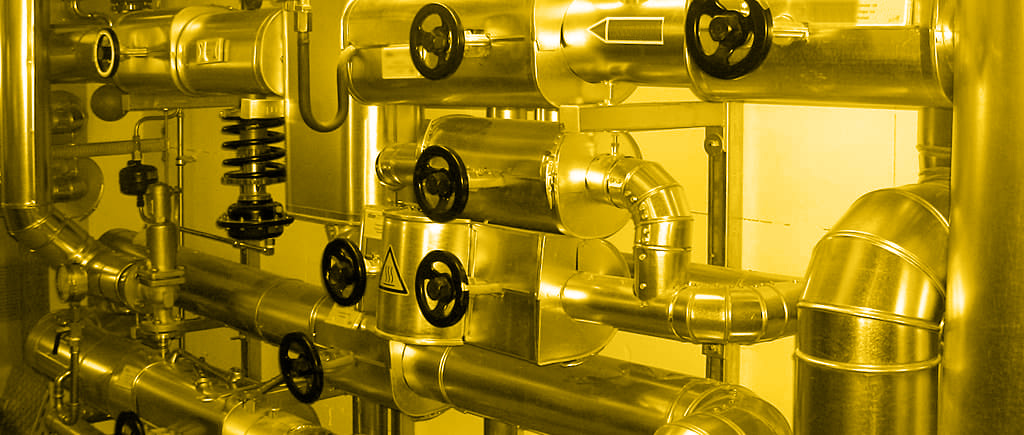# English

## Temperature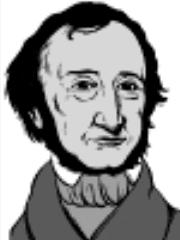Gabriel Fahrenheit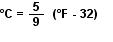Definition of Temperature. The degree of hotness with no implication of the amount of heat energy available. The temperature scale is used as an indicator of thermal equilibrium between two systems in contact with each other.

Temperature difference, as used in many heat transfer calculations, may be expressed in either °C or K. Since both scales have the same increments, a temperature difference of 1°C has the same value as a temperature difference of 1 K.

The Celsius (°C) scale. This is the scale most commonly used by the engineer, as it has a convenient (but arbitrary) zero temperature, corresponding to the temperature at which water will freeze.

The absolute or K (kelvin) scale. This scale has the same increments as the Celsius scale, but has a zero corresponding to the minimum possible temperature when all molecular and atomic motion has ceased. This temperature is often referred to as absolute zero (0 K) and is equivalent to -273.16°C.

The Fahrenheit (°F) scale. This scale is used in the FPS system(US and Canada), but not much elsewhere. To convert °F to °C, use the formula:

.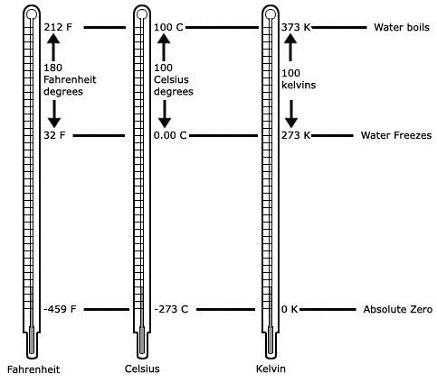Pic: Comparision of the various scales of temperature

## Pressure

Definition of Pressure is defined as 1 newton of force per square metre (1 N/m²). The SI unit of pressure is the pascal (Pa), but as Pa is such a small unit the kPa (1 kilonewton/m²) or MPa (1 Meganewton/m²) tend to be more appropriate to steam engineering.

However, probably the most commonly used metric unit for pressure measurement in steam engineering is the bar. This is equal to 105 N/m², and approximates to 1 atmosphere.

Absolute pressure (bar a)
This is the pressure measured from the datum of a perfect vacuum. So, a perfect vacuum has a pressure of 0 bar a.

The theoretical pressureless state of a perfect vacuum is "absolute zero". Absolute pressure is, therefore, the pressure above absolute zero.

At mean sea level, for instance, the pressure exerted by the atmosphere is 1.033 kg/cm2 absolute, when measured as kilograms per square centimeter. This is always assumed to be 1 kg/cm2 a for calculations.

At sea level, the pressure can also be stated as 1.013 25 bar a (1 atm) , when measured in bars. This is always assumed to be 1 bar a for calculations.

Absolute pressure is also commonly measured in millimeters of mercury, or "mm Hg".

Gauge pressure (bar g)
Bar gauge is the pressure as measured by the pressure gauge measured from the datum of the atmospheric pressure.Gauge pressure indication is shown as kg/cm2g.

The pressure gauge – bourdon tube type - measures pressure relative to the outside atmospheric pressure. This is rounded off to 1 bar a or 1 kg/cm2a – at (MSL). Therefore, to convert bar g to bar a, we add 1 bar, and to convert kg/cm2g to kg/cm2a, we again add 1 kg/cm2.

Gauge pressure + Atmospheric pressure = Absolute pressure
6 bar g + 1 bar = 7 bar a
10 kg/cm2g + 1 kg/cm2 = 11 kg/cm2a

Pressures above atmospheric will therefore, always yield a positive gauge pressure. Conversely a vacuum or negative pressure is the pressure below that of the atmosphere. A pressure of -1 bar g corresponds closely to a perfect vacuum. In the other units, a vacuum exists below zero kg/cm2g.

10 bar g = 11 bar a = 10.2 kg/cm2g = 11.2 kg/cm2a = 145 psig = 1 MPa = 106 N/m2

All the data given in the steam table has pressure in kg/cm2gauge and abs . You can check the steam tables and see that in the lower values for pressure, the enthalpy values vary a lot more than the higher pressure readings. Therefore it is very important you convert bar g to bar a, when the steam tables have pressures mentioned in bar terms.

Differential pressure ΔT
This is simply the difference between two pressures. When calculating difference in pressure, the reference point becomes meaningless. Therefore, the difference between two pressures will have the same value whether these pressures are measured in gauge pressure or absolute pressure, as long as the two pressures are measured from the same reference.

## Terms and Definitions

Thermodynamics
Thermodynamics is the study of energy changes accompanying physical and chemical changes. The term itself clearly suggests what is happening -- "thermo", from temperature, meaning energy, and "dynamics", which means the change over time. Thermodynamics can be roughly encapsulated with these topics:

Heat and Work / Energy / Enthalpy / Entropy / Free Energy

Energy
Energy is the capacity to do work (a translation from Greek -"work within"). Therefore work and energy are one and the same. The SI unit for work and energy is the joule, defined as 1 Nm.

The total energy of a system is composed of the internal, potential and kinetic energy. The temperature of a substance is directly related to its internal energy. The internal energy is associated with the motion, interaction and bonding of the molecules within a substance. The external energy of a substance is associated with its velocity and location, and is the sum of its potential and kinetic energy.

In physics, the amount of mechanical work done can be determined by an equation derived from Newtonian mechanics

work = opposing force x displacement

Where,
work is in joules (N*m) (or calories, but we are using primarily SI units)
opposing force is in newtons (kg*m/s2)
displacement is in meters

In chemical reactions, work is primarily related with expansion. It is generally defined as :

work = (area X applied pressure) X displacement

The value of displacement X area is actually the change in volume. If we imagine a reaction taking place in a container of some volume, we measure work by pressure times the change in volume.

w = dV x P

Where,
dV is the change in volume, in litres

Heat and Work
Heat and work are both forms of energy. They are also related forms, in that one can be transformed into the other. Heat energy (such as steam engines) can be used to do work (such as pushing a train down the track). Work can be transformed into heat, such as might be experienced by rubbing your hands together to warm them up.
Work and heat can both be described using the same unit of measure. Units of heat energy used may be calorie (cal), Joule (J, SI unit) or Btu.
Typically, we use the SI units of Joules (J) and kilojoules (kJ). But sometimes, the calorie is the unit of measure (MKS unit). Heat energy is measured in kilocalories, or 1000 calories.

Calorie
Calorie is defined as an amount of heat required to change temperature of one gram of liquid water by one degree Celsius.

1 cal = 4.186 J
1 kcal = 1000 cal = 4186.8 J

Joule
The joule is a derived unit defined as the work done or energy required, to exert a force of one newton for a distance of one metre, so the same quantity may be referred to as a newton metre or newton-metre with the symbol N·m.

One Joule is the mechanical energy which must be expended to raise the temperature of a unit weight (2 kg) of water from 0°C to 1°C, or from 32°F to 33°F.

1 J (Joule) = 2.389 X 10-4 kcal

Btu. A Btu - British thermal unit - is the amount of heat energy required to raise the temperature of one pound of cold water by 1º F. Or, a Btu is the amount of heat energy given off by one pound of water in cooling, say, from 70º F to 69º F.

Heat. Heat is energy transferred as a result of a temperature differences. Energy as heat passes from a warm body (with higher temperature) to a cold body (with lower temperature). Heat is a form of energy and as such is part of the enthalpy of a liquid or gas.

It is a measure of energy available with no implication of temperature. To illustrate , the one kcal that raises one kg of water from 18ºC to 19ºC could come from the surrounding air at a temperature of 35ºC or from a flame at a temperature of 1,500ºC.

Heat flow. The transfer of energy as a result of the difference in temperature alone is referred to as heat flow.

What is the difference btween temperature and Heat?
Temperature is the cause. Heat is the effect.

Watt is the SI unit of power and can be defined as 1 J/s of heat flow.

Heat Capacity. Heat Capacity of a system is the amount of heat required to change temperature of the whole system by one degree.

Specific Heat Capacity
A measure of the ability of a substance to absorb heat. It is the amount of energy (kcal) required to raise 1 kg of water 1° C. Thus specific heat capacity is expressed in kcal/kg/°C.
The specific heat capacity of water is 1 kcal/kg/° C. This means that an increase in enthalpy of 1 kcal will raise the temperature of 1 kg of water by 1° C.

Specific heat capacity. Specific heat, given by the symbol "C", is generally defined as:
The amount of heat required to raise the temperature of one (1) kilogram of a substance by one (1) degree.
Or,
The heat required to raise one (1) gram of a material one (1) degree. It can be thought of as the ability of a substance to absorb heat.

Water has a very large specific heat capacity (4.19 kJ/kg°C) or, 1 cal/gram°C compared with many fluids. Water is therefore, a good heat carrier.

Specific heat may be measured in kJ/kgC,kcal/kg°C, cal/gram°C or Btu/lb°F. For comparing units, check the unit converter for more information. Specific heat capacities for different materials can be found in Maths.

Amount of Heat Required to Rise Temperature
The amount of heat needed to heat a subject from one temperature level to an other can be expressed a

Cp =       Q
M X ∆T

Where,
Cp = specific heat capacity (kcal/kg°C)
Q = amount of heat added (kcal)
M = mass (kg)
ΔT = rise in temperature of the material in degrees Celsius (°C)

Heat Transfer
Heat transfer is the flow of enthalpy from matter at a high temperature to matter at a lower temperature when brought into contact.

Enthalpy
This is the term given to the total energy, due to both pressure and temperature, of a fluid (such as water or steam) at any given time and condition. More specifically it is the sum of the internal energy and the work done by an applied pressure.
The basic unit of measurement is the SI unit joule (J). Since one joule represents a very small amount of energy, it is usual to use kilojoules (kJ) (1000 Joules).
In MKS units, the basic unit of measurement for all types of energy is kcal/kg.

Specific Enthalpy
The specific enthalpy is a measure of the total energy (enthalpy) of a unit mass (1 kg), and the units are usually kJ/kg or kcal/kg.

Heat of the Liquid (Enthalpy of Saturated Water) - hf
Expressed in kcal's, this is the amount of heat required to raise the temperature of 1 kg of water from 0° C to the boiling point of a given pressure/temperature correlation. Also referred to as Sensible Heat.

Written as hf – heat of the fluid.

Latent Heat of Evaporation (Enthalpy of Evaporation) - hfg
Expressed in kcal's, this is the amount of heat required to change 1 kg of boiling water to 1 kg of steam. This same amount of heat is released when a kg of steam is condensed back to a kg of water. The quantity of latent heat will vary with the pressure and/or temperature of a closed system.

Written as hfg – heat incurred in change of state from fluid to gas, or back.

Total Heat of Steam (Enthalpy of Saturated Steam) - hg
The sum of the Heat of the Liquid and Latent Heat of Evaporation, also expressed in kcal's.

Written as hg – Total enthalpy of saturated steam.

So,
Subscript f = Fluid or liquid state, for example hf: liquid enthalpy
Subscript fg = Change of state liquid to gas, for example hfg: enthalpy of evaporation
Subscript g = Total, for example hg: total enthalpy

Density and specific volume
The density ρ of a substance can be defined as its mass (m) per unit volume (V). The specific volume (vg) is the volume per unit mass and is therefore the inverse of density. In fact, the term ‘specific’ is generally used to denote a property of a unit mass of a substance.

Where,
ρ = Density(kg/m3)
m = Mass (kg)
V = Volume (m3)
vg = Specific volume (m3/kg)

The SI units of density (ρ) are kg/m³, whilst conversely the units of specific volume (Vg) are m³/kg.

Specific gravity

Another term used as a measure of density is the specific gravity. It is a ratio of the density of a substance (ρs) and the density of pure water (ρw) at standard temperature and pressure (STP). This reference condition is usually defined as being at atmospheric pressure and 0°C. Sometimes it is said to be at 20°C or 25°C and is referred to as normal temperature and pressure (NTP).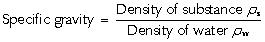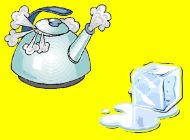The density of water at these conditions is approximately 1 000 kg/m³. Therefore substances with a density greater than this value will have a specific gravity greater than 1, whereas substances with a density less than this will have a specific gravity of less than 1.

Since the specific gravity is a ratio of two densities, it is a dimensionless variable and has no units. Therefore in this case the term specific does not indicate it is a property of a unit mass of a substance. The specific gravity is also sometimes known as the relative density of a substance.

Saturation Temperature (boiling point). The temperature for a corresponding Saturation Pressure at which a liquid boils into its vapor phase. The liquid can be said to be saturated with thermal (heat) energy. Any addition of thermal energy results in a phase change.

Boiling Point. A somewhat clearer (and perhaps more useful) definition of boiling point is "the temperature at which the vapor pressure of the liquid equals the pressure of the surroundings".

Saturation pressure. The pressure at which vaporization (boiling) starts to occur for a corresponing Saturation temperature. For water at 100°C, the saturation pressure is 1 atm and, for water at 1 atm, the saturation temperature is 100°C.

The term 'saturation' defines a condition in which a mixture of vapor and liquid can exist together at a given temperature and pressure.

## Quick reference

SI and other units
Temperature – °C or K

Pressure SI unit – Pascal
1 Pa = 1 N/m2, too small a unit
Common unit = Bar
1 Bar =105N/m2 = 0.1 Mpa

Atmospheric Pressure = 1 Bar abs at MSL
Vacuum = 0 Bar abs

Bar Gauge + atm pressure = Bar abs
barg = kg/cm2g

Density in kg/m3
Specific Volume = 1 / Density in m3/kg
Specific Gravity = Density ratio to water

Energy SI unit = 1 Joule = 1 Nm = 4.186 cal
Common unit = kilocalorie
1 kcal = heat reqd to raise 1 kg water by 1°C
1 kcal = 4186.8 Joules
Cp = sp. heat capacity in kcal/kg °C

Conversions between SI and other units

mWC = meters water column
1 Bar = 10 mWC

1 Bar = 14.23 PSI (Lbs/in2
150 psi = 10.54 Kg/cm2g
50 psi = 3.5 Kg/cm2g
• Head is the pressure exerted by a static head of water column. Remember that 10 meters of head = 1 bar
• Gauge pressure + Atmospheric pressure = Absolute pressure
• The most common pressure for utilization of steam is 3.5 kg/cm2g.
• Steam Density is about a thousandth of water.
• Boilers come from an era when the industrial revolution was at its peak in Britain. Therefore, the world standards for boilers are British. ie, the MKS system.
• Specific gravity is dimensionless as it is a ratio. Density of any lquid relative to water. It is used mostly for fuel.
• The SI unit of 1 Joule is too small, therefore the kilocalorie was developed.

## Unit converter

SI base units. The International System of Units (SI) is founded on seven base units: Length, Mass, Time, Electrical current, Thermodynamic temperature, Luminous temperature and Amount of substance. They are defined in an absolute way without referring to any other units. We will be working with the following four units only.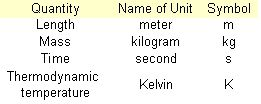Sl derived units. Derived units are algebraic combinations of the seven base units with some of the combinations being assigned special names and symbols.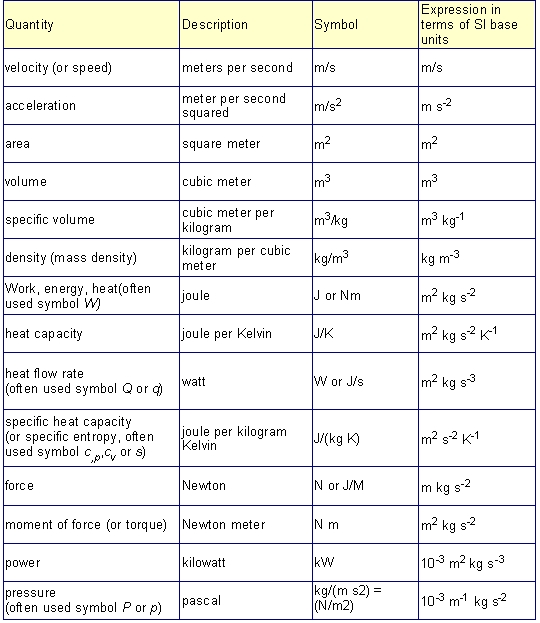All properties of matter are measured at STP - Standard temperatureand pressure.
Temperature: freezing point of pure water, 0°C or 273.15°K
Pressure: 760 mm Hg or one atmosphere

## Examples

Example 1. Heating Water
What is the energy needed to heat a mass of 1.0 kg of water from 0°C to 100°C when the specific heat of water is 1kcal/kg°C.
Q = M Cp ΔT
= 1 kg X 1 (kcal/kg°C) X (100 - 0)(°C)
= 100 kcal

Example 2.
If 200 kgs of a substance at 22 °C with a specific heat of 0.88 kcal/kg°C is heated with 10,000 kcal of energy, what is the new temperature of the substance?
Q = M Cp ΔT
ΔT = Q / M Cp

= 10,000 / 200 X 0.88 = 56.82
So, new temperature is 22+56.82 = 78.82°C

Example 3.
Assume that water at 50° C water is fed to a boiler at atm pressure. This begins to boil at 100° C. 1 kcal will be required to raise each kg of water by 1° C. Therefore, for each kg of water, the increase in enthalpy required to raise the temperature from 50° C to 100° C is:
(100 - 50) x 1 = 50 kcal/kg

If the boiler holds 10000 kg mass the increase in enthalpy to bring the total mass of water to it's boiling point is therefore:
50 kcal/kg x 10000 kg or 5,00,000 kcal.

It must be remembered, this figure is not the sensible heat, but merely the increase in sensible heat required to raise the temperature from 50° C to 100° C. The datum point of the steam tables is water at 0° C, which is assumed to have a heat content of zero for our purposes.

The total sensible heat of water at 100° C is therefore:

(100 - 0) x 1 = 100 kcal/kg